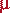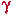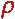Math & Science Home | Proficiency Tests | Mathematical Thinking in Physics | Aeronauts 2000

 CONTENTS Introduction Fermi's Piano Tuner Problem How Old is Old? If the Terrestrial Poles were to Melt... Sunlight Exerts Pressure Falling Eastward What if an Asteroid Hit the Earth Using a Jeep to Estimate the Energy in Gasoline How do Police Radars really work? How "Fast" is the Speed of Light? How Long is a Light Year? How Big is a Trillion? "Seeing" the Earth, Moon, and Sun to Scale Of Stars and Drops of Water If I Were to Build a Model of the Cosmos... A Number Trick Designing a High Altitude Balloon Pressure in the Vicinity of a Lunar Astronaut Space Suit due to Outgassing of Coolant Water Calendar Calculations Telling Time by the Stars - Sidereal Time Fields, an Heuristic Approach The Irrationality ofThe Irrationality ofThe Number (i)i Estimating the Temperature of a Flat Plate in Low Earth Orbit Proving that (p)1/n is Irrational when p is a Prime and n>1 The Transcendentality ofIdeal Gases under Constant Volume, Constant Pressure, Constant Temperature and Adiabatic Conditions Maxwell's Equations: The Vector and Scalar Potentials A Possible Scalar Term Describing Energy Density in the Gravitational Field A Proposed Relativistic, Thermodynamic Four-Vector Motivational Argument for the Expression-eix=cosx+isinx Another Motivational Argument for the Expression-eix=cosx+isinx Calculating the Energy from Sunlight over a 12 hour period Calculating the Energy from Sunlight over actual full day Perfect Numbers-A Case Study Gravitation Inside a Uniform Hollow Sphere Further note on Gravitation Inside a Uniform Hollow Sphere Pythagorean Triples Black Holes and Point Set Topology Additional Notes on Black Holes and Point Set Topology Field Equations and Equations of Motion (General Relativity) The observer in modern physics A Note on the Centrifugal and Coriolis Accelerations as Pseudo Accelerations - PDF File On Expansion of the Universe - PDF File

## Ideal Gases under Constant Volume, Constant Pressure, Constant Temperature, & Adiabatic Conditions

Note to the student: The following section is a reduction of college notes I made in introductory thermodynamics. It does not read as easily as the preceding sections. I include it here because, for me, it represented a significant unification of the ideas presented in the text and during lecture. The first year college student will certainly find it useful.

The equation of state for an ideal gas is

 pV =RT 1

where p is gas pressure, V is volume,is the number of moles, R is the universal gas constant (= 8.3144 j/(oK mole)), and T is the absolute temperature. The first law of thermodynamics, the conservation of energy, may be written in differential form as

 dq = du + p dV 2

where dq is a thermal energy input to the gas, du is a change in the internal energy of the gas, and p dV is the work done by the gas in expanding through the change in volume dV.

Constant Volume Process

If V = const., then dV = 0, and, from 2, dq = du; i.e., all the thermal input to the gas goes into internal energy of the gas. We should expect a temperature rise. If the gas has a specific heat at constant volume of CV (j/(oK mole)), then we may set dq =CV dT. It follows, in this case, that

 du =CV dT 3

Since du was initially unspecified, we are free to choose its mathematical form. Equation 2 will be retained for du throughout the remainder of the cases.

Constant Pressure Process

If p = const., then dp = 0, and, from 1, p dV =R dT; i.e., the work done by the gas in expanding through the differential volume dV is directly proportional to the temperature change dT. If the gas has a specific heat at constant pressure of Cp, then dq =Cp dT, and, from 2 (with 3),Cp dT =CV dT +R dT

Simplifying gives an important constitutive relationship between CV, Cp, and R, namely:

 Cp = CV + R 4

Constant Temperature Process

If T = const., then dT = 0, and, from 1, d(pV) = 0, i.e., pressure and volume are inversely proportional. Further, from 2, dq = p dV; i.e., there is no change in internal energy (from 3, du = 0), and all the thermal input to the gas goes into the work of expansion.

If q = const, then dq = 0, and, from 2 (with 3), 0 =CV dT + p dV; i.e., internal energy of the gas might be reduced in favor of expansion, or vice versa. This expression may be written in an equivalent form as

 0 = (CV/R)(dT/T) + dV/V 5

(division of the first term byRT, and the second term by pV). Further, from 1,

p dV + V dp =R dT
 or, equivalently, dp/p + dV/V = dT/T 6

(division of the Left Hand Side by pV, and the Right Hand Side byRT).

Equations 5 and 6 may be used to develop relationships between p and V, or p and T:

Case 1:

To eliminate T, use 6 in 5 for dT/T to obtain 0 = (CV/R)(dp/p + dV/V) + dV/V, or

 -(CV/R) dp/p = (1 + CV/R) dV/V 6a.

Using 4, we may write CV/R = CV/(Cp - CV) = 1/(- 1) where= Cp/CV, the ratio of the specific heats (>1; in fact, approximately 1.4 for air at STP). Thus, equation 6a becomes (after simplification)

-dp/p =dV/V

which, upon integration, yields

 p0/p = (V/V0)7

i.e., the pressure varies inversely as the volume raised to the power.

Case 2:

To eliminate V, use equation 6 to write dV/V = dT/T - dp/p, and substitute for dV/V in eq. 5

(CV/R + 1) dT/T = dp/p

Proceeding as before produces the result that/(- 1) p/p0 = (T/T0) 8.

Entropy changes may be calculated for each of the above thermodynamic processes. The definition of entropy is

 dS = dq/T 9

where dS is the differential entropy change.

For constant volume processes, dq =CV dT, so that dS =CV dT/T, andS =CV ln(T/T0) 10

For constant pressure processes, dq =Cp dT, so that dS =Cp dT/T, andS =Cp ln(T/T0) 11

For constant temperature processes, dq = p dV, so that dS = p dV/T =R dV/V, andS =R ln(V/V0) 12

For adiabatic processes, dq = 0, so that ds = 0, and

S = const.

Problem:
Estimate the dry adiabatic lapse rate for an ascending parcel of air near the earth's surface.

Solution:
The dry adiabatic lapse rate for an ascending parcel of air near the earth's surface may be estimated from the above expressions. (For comparison, the published value is 5.5 oF per thousand feet). Let us begin with Bernoulli's equation

 p +gh = p0 13

where p is the pressure of air at height h above the earth's surface,is the density of air in kg/m3, g is the gravitational acceleration, and p0 is atmospheric pressure at the earth's surface. Differentiating this expression once, we get

 dp +g dh = 0 13a.

We now write the equation of state for an ideal gas as p = nkT, where n is the number density of the gas in m-3, and k is Boltzmann's constant. We may further write n =/M where M is the average molecular mass of air in kg. Using this expression in the ideal gas equation of state and solving for, we have= pM/kT. Substituting this result into 13a gives

dp + (pMg/kT) dh = 0

We may now separate variables and integrate. In so doing, it is customary to assume that the variation of T with h may be ignored (isothermal approximation). Thus

dp/p = -(Mg/kT) dh
 or p/p0 = e-(Mg/kT)h 14

The term kT/Mg is often called the scale height of the atmosphere (i.e., that height at which the calculated pressure drops to 1/e of its initial value; in an isothermal atmosphere, this number has physical meaning). At the earth's surface, at STP (Standard Temperature and Presure, 1 atm.), we have

M = 4.81 x 10-26 kg
g = 9.81 m/sec2
k = 1.38 x 10-23 j/oK
T = 273 oK

so that the scale height is 7.99 km = 2.53 x 104 ft. If we now set p0 = 1 atm, then ath = 1,000 ft., we find

p = 9.61 x 10-1 atm.

We may use this pressure in equation 8 as an estimate of atmospheric pressure (even though eq. 8 pertains to an adiabatic atmosphere rather than an isothermal one), and calculate the temperature at 1,000 ft. When we do so, we find

T = 270 oK
 orT = 3 oK = 5.4 oF 15

This value is satisfactorily close to the book value of 5.5 oF per 1,000 ft. to meet our needs.